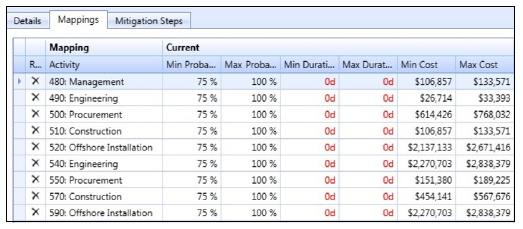# The Basics Behind Cost Risk Analysis

Posted by Megan Cacioppo on July 20, 2017If you’re familiar with schedule risk analysis, you’re already well on your way to understanding the basics behind cost risk analysis.  Because at their core, both processes are very similar.  In a schedule risk analysis you define schedule uncertainty, then identify and map schedule risk events.  In a cost risk analysis, you do the almost exactly the same thing, but with… you guessed it, costs.

Let’s dig in a little deeper.

Step 1: Identify the Base Cost Uncertainty

The first step in cost risk analysis is to identify the base cost uncertainty. As with schedule risk exposure, total cost risk exposure is divided into two buckets: cost uncertainty and cost discrete risk events. And also similar to schedule risk, it’s important when you’re setting up the risk model that you put the right things into the right buckets, so items aren’t missed and aren’t double‐dipped.

Cost uncertainty has two main components: base cost uncertainty and the uncertainty contribution due to time. You must account for both of these components in the cost uncertainty, and its best if you can do so independently of each other. Including and analyzing them separately makes it easier to determine where to spend time and effort. One helpful tip is to organize the cost estimate with separate lines for time independent and time‐dependent costs. This way of organizing will make the assembly of the cost risk model a whole lot easier. It also makes both the model and the analysis more accurate and precise.

Step 2: Determine the Cost Risk Events

The next step in the process is to quantify and assign the discrete cost risk events in the model. Just like setting up schedule risk events, the discrete cost risk events are captured in the risk register. Frequently, the discrete schedule risk events and the discrete cost risk events are the same. The risk matrix used for the cost risk analysis can and should be the same as the matrix used for the schedule risk analysis. You do, however, need to evaluate the cost impact range for accuracy and appropriateness.

Similarly, the information contained in the risk register is no different for cost risk events as it is for schedule risk events. You’ll see the same risk event base information, mitigation actions, and post‐mitigation ratings. After the cost risk events are captured in the risk register, you must quantify both the probability and the impact. This leads to the calculation of the overall risk score (which should not be used).

Step 3: Cost Risk Mapping

The last step before running the cost risk analysis is to attach the risk events to whatever line or lines they will impact if they happen. As with the schedule work, this is called mapping the risks. Check out the figure below to see an example of risk mapping in action:Step 4: Analyze

Now you’re ready to analyze, analyze, analyze and get to the bottom of cost issues that otherwise might be surprises, and decide what to do about them. But which analyses should you run and why? Check out Chapter 6 of our new eBook, Project Risk Management for Dummies to learn more about which analyses you should run to determine cost risk exposure, and how you can then compare them to learn how much risk comes from uncertainties and how much from risk events.

Subscribe >>